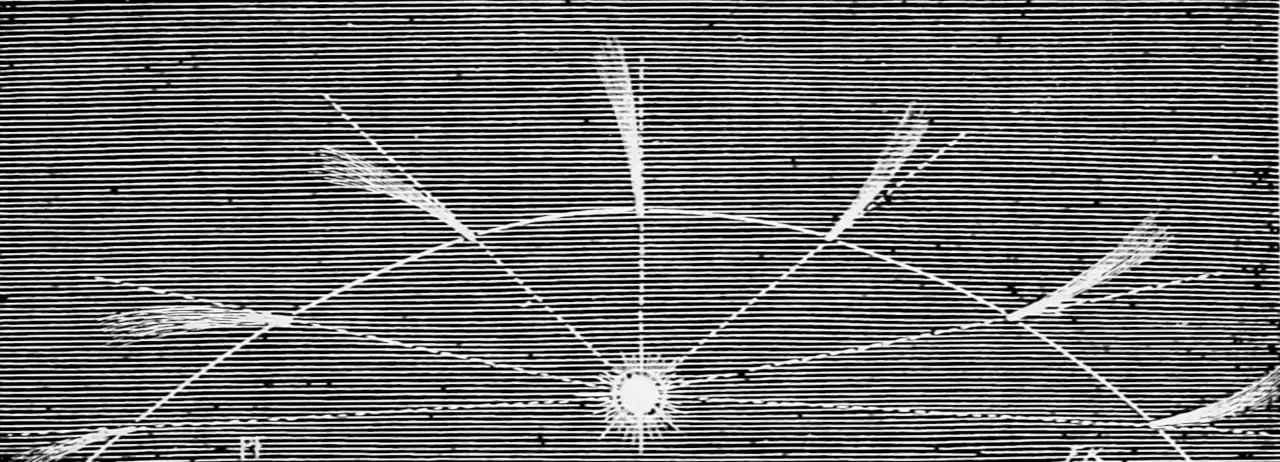# Transforms of random variatesI have a nonlinear transformation of a random process. What is its distribution?

Related: What is the gradient of the transform? That is the topic of the reparameterization trick and other MC grad estimators. This is very commonly seen in the context of transforms of Gaussians when it produces results like the delta method and extended Kalman filtering.

## Taylor expansion

Not complicated but subtle .

Consider a general nonlinear differentiable transformation $$g$$ and its second order Taylor expansion. Consider the mapping $$g:\mathbb{R}^{n_{x}}\to\mathbb{R}^{n_{z}}$$ applied to a variable $$x,$$ defining $$z:=g(x).$$ Let $$\mathrm{E}(x)=\mu_{x}$$ and $$\operatorname{Var}(x)=P_{x}.$$ The Hessian of the $$i^{\text {th }}$$ component of $$g$$ is denoted $$g_{i}^{\prime \prime}.$$ $$[x_i]_i$$ is a vector where the $$i$$th element is $$x_i$$. We will approximate $$z$$ using the Taylor expansion, $z=g\left(\mu_{x}\right)+g^{\prime}\left(\mu_{x}\right)\left(x-\mu_{x}\right)+\left[\frac{1}{2}\left(x-\mu_{x}\right)^{T} g_{i}^{\prime \prime}\left(\mu_{x}\right)\left(x-\mu_{x}\right)\right]_{i}.$ Leaving aside questions of when this is convergent for now. Then the first moment of $$z$$ is given by $\mu_{z}=g\left(\mu_{x}\right)+\frac{1}{2}\left[\operatorname{tr}\left(g_{i}^{\prime \prime}\left(\mu_{x}\right) P_{x}\right)\right]_{i}$ Further, let $$x \sim \mathcal{N}\left(\mu_{x}, P_{x}\right)$$, then the second moment of $$z$$ is given by $P_{z}=g^{\prime}\left(\mu_{x}\right) P_{x}\left(g^{\prime}\left(\mu_{x}\right)\right)^{T}+\frac{1}{2}\left[\operatorname{tr}\left(g_{i}^{\prime \prime}\left(\mu_{x}\right) P_{x} g_{j}^{\prime \prime}\left(\mu_{x}\right) P_{x}\right)\right]_{i j}$ with $$i, j=1, \ldots, n_{z}.$$

This is commonly seen in the context of transforms of Gaussians.

## Unscented transforms

Typically seen for Gaussian RVs. Ebeigbe et al. (2021) claims to have devised a method for more general RVs.

## Stein’s lemma

As seen in Stein’s method. Gives us the special case of certain exponential RVS (typically Gaussian) under certain matched transforms. Long story.

## Stochastic Itô-Taylor expansion

Taylor expansions for stochastic processes. See stochastic taylor expansion. tl;dr: Usually more trouble than it is worth.

## Learnable transforms

### No comments yet. Why not leave one?

GitHub-flavored Markdown & a sane subset of HTML is supported.